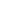## Probability theoryProbability theory is the branch of mathematics concerned with probability, the analysis of random phenomena. The central objects of probability theory are random variables, stochastic processes, and events: mathematical abstractions of non-deterministic events or measured quantities that may either be single occurrences or evolve over time in an apparently random fashion.

If an individual coin toss or the roll of dice is considered to be a random event, then if repeated many times the sequence of random events will exhibit certain patterns, which can be studied and predicted. Two representative mathematical results describing such patterns are the law of large numbers and the central limit theorem.
As a mathematical foundation for statistics, probability theory is essential to many human activities that involve quantitative analysis of large sets of data. Methods of probability theory also apply to descriptions of complex systems given only partial knowledge of their state, as in statistical mechanics. A great discovery of twentieth century physics was the probabilistic nature of physical phenomena at atomic scales, described in quantum mechanics.
Theory Explanation:
The mathematical theory of probability has its roots in attempts to analyze games of chance by Gerolamo Cardano in the sixteenth century, and by Pierre de Fermat and Blaise Pascal in the seventeenth century (for example the "problem of points"). Christiaan Huygens published a book on the subject in 1657 and in the 19th century a big work was done by Laplace in what can be considered today as the classic interpretation.
Initially, probability theory mainly considered discrete events, and its methods were mainly combinatorial. Eventually, analytical considerations compelled the incorporation of continuous variables into the theory.
This culminated in modern probability theory, on foundations laid by Andrey Nikolaevich Kolmogorov. Kolmogorov combined the notion of sample space, introduced by Richard von Mises, and measure theory and presented his axiom system for probability theory in 1933. Fairly quickly this became the mostly undisputed axiomatic basis for modern probability theory but alternatives exist, in particular the adoption of finite rather than countable additivity by Bruno de Finetti.
Most introductions to probability theory treat discrete probability distributions and continuous probability distributions separately. The more mathematically advanced measure theory based treatment of probability covers both the discrete, the continuous, any mix of these two and more.

Labels:
•    Type→ Basic Science and social sciencce
•    Theorist→ Blaise Pascal
•    Date →1654

### Online visitor

We have 62 guests and no members online